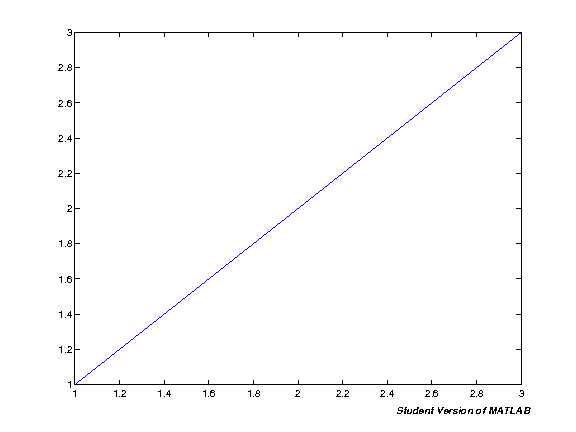Snippets (MATLAB)

My book Create Simple GUI Applications with Python & Qt5 has been updated! New chapters on the Model View architecture and custom widgets.

This notebook contains snippets of code that are useful when working with MATLAB in IPython Notebooks.

Displaying images from MATLAB¶

Passing variables into cells using the %%matlab magic requires h5py which is tricky to install. If you only need to pass in simple variables (strings) you can instead pass it in using Python string formatting and mlab.run_code. Unfortunately, this means we don't benefit from pymatbridge automatically rendering figures. The class below is just a helper Python class to make it simpler to show the resulting figures from MATLAB commands.

In :
import base64, os

os.environ['http_proxy'] = ''

class ImageOut(object):
def __init__(self, img):
with open(img) as f:
self.image = base64.b64encode(data)
def _repr_png_(self):
return self.image
In :
from pymatbridge import Matlab
mlab = Matlab()
mlab.start()
r = mlab.run_code('''
plot([1,2,3]);
''')
ImageOut( r['content']['figures'] )
Starting MATLAB on http://localhost:60894
visit http://localhost:60894/exit.m to shut down same
.....MATLAB started and connected!
Out:Pass widget variables into %%matlab magic¶

If you want to use widget controls in IPython notebooks you might find yourself wanting to pass those values into matlab cells using %%matlab magic. This snippet shows how you can use a callback function together with interact to automatically update global vars, and then pass these into the matlab session using -i.

Note: you need hdf5 and h5py installed for this to work

In :
Starting MATLAB on http://localhost:60938
visit http://localhost:60938/exit.m to shut down same
...MATLAB started and connected!
In :
def widget_callback(**kwargs):
for k,v in kwargs.items():
globals()[k] = v
In :
myvar=5

Note: We define the variable first above, and pass the output var in as an default value. This means the widget wont reset if the cell is re-run, but will instead keep the current value. To reset the value just run the cell above.

In :
from IPython.html.widgets import interact
from IPython.html import widgets

i = interact(widget_callback,
myvar=widgets.IntSliderWidget(min=1, max=50, step=1, value=myvar, description="Reference spc:"),
)

In :
%%matlab -i myvar
myvar
myvar =
13
In [ ]: